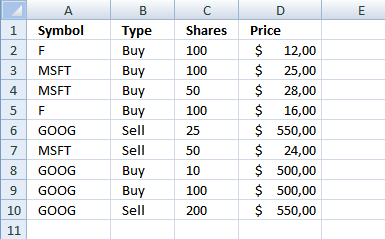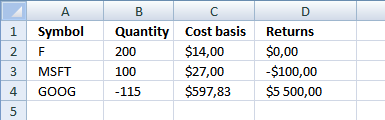Author: Oscar Cronquist Article last updated on October 30, 2018

This is follow up post to: Tracking a stock portfolio in excel (auto update)
In this post we are going to calculate cost basis and returns. The calculations are simplified, commissions, stock splits and dividends are removed from calculations.

In the first post we created dynamic ranges.We also identified accumulated stocks and the number of shares. I have now added cost basis and returns.Excel formula in cell C2:

Copy cell C2 and paste down as far as needed.

How this formula works in cell C2

Step 1 - Calculate total cost you paid

SUMPRODUCT(array1, array2, )
Returns the sum of the products of the corresponding ranges or arrays

becomes

becomes

SUMPRODUCT(({TRUE, FALSE, FALSE, TRUE, FALSE, FALSE, FALSE, FALSE, FALSE}))*({TRUE, TRUE, TRUE, TRUE, FALSE, FALSE, TRUE, TRUE, FALSE})*{100, 100, 50, 100, 25, 50, 10, 100, 200}*{12, 25, 28, 16, 550, 24, 500, 500, 550})

becomes

SUMPRODUCT({1200, 0, 0, 1600, 0, 0, 0, 0, 0}) returns 2800.

Step 2 - Calculate total amount you sold

SUMPRODUCT((A2=Symbol)*(Type="Sell")*Shares*Price)

becomes

SUMPRODUCT({0, 0, 0, 0, 0, 0, 0, 0, 0}) returns 0.

Step 3 - Calculate accumulated shares

becomes

SUMPRODUCT({100, 0, 0, 100, 0, 0, 0, 0, 0}) - SUMPRODUCT({0, 0, 0, 0, 0, 0, 0, 0, 0})

and returns 200.

Step 4 - Calculate cost basis

becomes

=IF(A2<>"", (2800-0)/200, "") returns 14 in cell C2.Excel formula in cell D2

=IF(A2<>"", (IFERROR(SUMPRODUCT(--(A2=Symbol), --("Sell"=Type), Price, Shares)/SUMPRODUCT(--(A2=Symbol), --("Sell"=Type), Shares), 0)-IFERROR(SUMPRODUCT(--(A2=Symbol), --("Buy"=Type), Price, Shares)/SUMPRODUCT(--(A2=Symbol), --("Buy"=Type), Shares), 0))*(MIN(SUMPRODUCT(--(A2=Symbol), --("Sell"=Type), Shares), SUMPRODUCT(--(A2=Symbol), --("Buy"=Type), Shares))), "")

Copy cell D2 and paste down as far as needed.

How this formula works in cell D2

Step 1 - Calculate average price you paid per share

=IF(A2<>"", (IFERROR(SUMPRODUCT(--(A2=Symbol), --("Sell"=Type), Price, Shares)/SUMPRODUCT(--(A2=Symbol), --("Sell"=Type), Shares), 0)-IFERROR(SUMPRODUCT(--(A2=Symbol), --("Buy"=Type), Price, Shares)/SUMPRODUCT(--(A2=Symbol), --("Buy"=Type), Shares), 0))*(MIN(SUMPRODUCT(--(A2=Symbol), --("Sell"=Type), Shares), SUMPRODUCT(--(A2=Symbol), --("Buy"=Type), Shares))), "")

(IFERROR(SUMPRODUCT(--(A2=Symbol), --("Sell"=Type), Price, Shares)/SUMPRODUCT(--(A2=Symbol), --("Sell"=Type), Shares), 0)

returns 0.

Step 2 - Calculate average selling price

=IF(A2<>"", (IFERROR(SUMPRODUCT(--(A2=Symbol), --("Sell"=Type), Price, Shares)/SUMPRODUCT(--(A2=Symbol), --("Sell"=Type), Shares), 0)-IFERROR(SUMPRODUCT(--(A2=Symbol), --("Buy"=Type), Price, Shares)/SUMPRODUCT(--(A2=Symbol), --("Buy"=Type), Shares), 0))*(MIN(SUMPRODUCT(--(A2=Symbol), --("Sell"=Type), Shares), SUMPRODUCT(--(A2=Symbol), --("Buy"=Type), Shares))), "")

returns 14.

Step 3 - Multiply with bought or sold shares

=IF(A2<>"", (IFERROR(SUMPRODUCT(--(A2=Symbol), --("Sell"=Type), Price, Shares)/SUMPRODUCT(--(A2=Symbol), --("Sell"=Type), Shares), 0)-IFERROR(SUMPRODUCT(--(A2=Symbol), --("Buy"=Type), Price, Shares)/SUMPRODUCT(--(A2=Symbol), --("Buy"=Type), Shares), 0))*(MIN(SUMPRODUCT(--(A2=Symbol), --("Sell"=Type), Shares), SUMPRODUCT(--(A2=Symbol), --("Buy"=Type), Shares))), "")

MIN(SUMPRODUCT(--(A2=Symbol), --("Sell"=Type), Shares), SUMPRODUCT(--(A2=Symbol), --("Buy"=Type), Shares))

returns 0.

Step 4 - Calculate returns

=IF(A2<>"", (IFERROR(SUMPRODUCT(--(A2=Symbol), --("Sell"=Type), Price, Shares)/SUMPRODUCT(--(A2=Symbol), --("Sell"=Type), Shares), 0)-IFERROR(SUMPRODUCT(--(A2=Symbol), --("Buy"=Type), Price, Shares)/SUMPRODUCT(--(A2=Symbol), --("Buy"=Type), Shares), 0))*(MIN(SUMPRODUCT(--(A2=Symbol), --("Sell"=Type), Shares), SUMPRODUCT(--(A2=Symbol), --("Buy"=Type), Shares))), "")

becomes

=IF(A2<>"", (0-14)*0) returns 0

Final notes

I am not telling you to sell or buy any stock, this is just an example.

I hope I got all calculations right.

Get excel file

Tracking-a-stock-portfolio2.xlsx
(Excel 2007 -2010 Workbook *.xlsx)